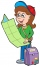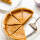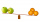# Fraction calculator

The calculator performs basic and advanced operations with fractions, expressions with fractions combined with integers, decimals, and mixed numbers. It also shows detailed step-by-step information about the fraction calculation procedure. Solve problems with two, three, or more fractions and numbers in one expression.

## Result:

### 34/5 * 15/7 = 228/35 = 6 18/35 ≅ 6.5142857

Spelled result in words is two hundred twenty-eight thirty-fifths (or six and eighteen thirty-fifths).

### How do you solve fractions step by step?

1. Conversion a mixed number 3 4/5 to a improper fraction: 3 4/5 = 3 4/5 = 3 · 5 + 4/5 = 15 + 4/5 = 19/5

To find a new numerator:
a) Multiply the whole number 3 by the denominator 5. Whole number 3 equally 3 * 5/5 = 15/5
b) Add the answer from previous step 15 to the numerator 4. New numerator is 15 + 4 = 19
c) Write a previous answer (new numerator 19) over the denominator 5.

Three and four fifths is nineteen fifths
2. Conversion a mixed number 1 5/7 to a improper fraction: 1 5/7 = 1 5/7 = 1 · 7 + 5/7 = 7 + 5/7 = 12/7

To find a new numerator:
a) Multiply the whole number 1 by the denominator 7. Whole number 1 equally 1 * 7/7 = 7/7
b) Add the answer from previous step 7 to the numerator 5. New numerator is 7 + 5 = 12
c) Write a previous answer (new numerator 12) over the denominator 7.

One and five sevenths is twelve sevenths
3. Multiple: 19/5 * 12/7 = 19 · 12/5 · 7 = 228/35
Multiply both numerators and denominators. Result fraction keep to lowest possible denominator GCD(228, 35) = 1. In the following intermediate step, the fraction result cannot be further simplified by canceling.
In other words - nineteen fifths multiplied by twelve sevenths = two hundred twenty-eight thirty-fifths.

#### Rules for expressions with fractions:

Fractions - use the slash “/” between the numerator and denominator, i.e., for five-hundredths, enter 5/100. If you are using mixed numbers, be sure to leave a single space between the whole and fraction part.
The slash separates the numerator (number above a fraction line) and denominator (number below).

Mixed numerals (mixed fractions or mixed numbers) write as non-zero integer separated by one space and fraction i.e., 1 2/3 (having the same sign). An example of a negative mixed fraction: -5 1/2.
Because slash is both signs for fraction line and division, we recommended use colon (:) as the operator of division fractions i.e., 1/2 : 3.

Decimals (decimal numbers) enter with a decimal point . and they are automatically converted to fractions - i.e. 1.45.

The colon : and slash / is the symbol of division. Can be used to divide mixed numbers 1 2/3 : 4 3/8 or can be used for write complex fractions i.e. 1/2 : 1/3.
An asterisk * or × is the symbol for multiplication.
Plus + is addition, minus sign - is subtraction and ()[] is mathematical parentheses.
The exponentiation/power symbol is ^ - for example: (7/8-4/5)^2 = (7/8-4/5)2

#### Examples:

subtracting fractions: 2/3 - 1/2
multiplying fractions: 7/8 * 3/9
dividing Fractions: 1/2 : 3/4
exponentiation of fraction: 3/5^3
fractional exponents: 16 ^ 1/2
adding fractions and mixed numbers: 8/5 + 6 2/7
dividing integer and fraction: 5 ÷ 1/2
complex fractions: 5/8 : 2 2/3
decimal to fraction: 0.625
Fraction to Decimal: 1/4
Fraction to Percent: 1/8 %
comparing fractions: 1/4 2/3
multiplying a fraction by a whole number: 6 * 3/4
square root of a fraction: sqrt(1/16)
reducing or simplifying the fraction (simplification) - dividing the numerator and denominator of a fraction by the same non-zero number - equivalent fraction: 4/22
expression with brackets: 1/3 * (1/2 - 3 3/8)
compound fraction: 3/4 of 5/7
fractions multiple: 2/3 of 3/5
divide to find the quotient: 3/5 ÷ 2/3

The calculator follows well-known rules for order of operations. The most common mnemonics for remembering this order of operations are:
PEMDAS - Parentheses, Exponents, Multiplication, Division, Addition, Subtraction.
BEDMAS - Brackets, Exponents, Division, Multiplication, Addition, Subtraction
BODMAS - Brackets, Of or Order, Division, Multiplication, Addition, Subtraction.
GEMDAS - Grouping Symbols - brackets (){}, Exponents, Multiplication, Division, Addition, Subtraction.
Be careful, always do multiplication and division before addition and subtraction. Some operators (+ and -) and (* and /) has the same priority and then must evaluate from left to right.

## Fractions in word problems:

• Stones in aquariumIn an aquarium with a length 2 m; width 1.5 m and a depth of 2.5 m is a water level up to three-quarters of the depth. Can we place stones with a volume of 2 m3 into the aquarium without water being poured out?
• Engineer KažimírThe difference between politicians-demagogues and reasonable person with at least primary education beautifully illustrated by the TV show example. "Engineer" Kažimír says that during their tenure there was a large decline in the price of natural gas, pri
• Comparing and sortingArrange in descending order this fractions: 2/7, 7/10 & 1/2
• OrchardOne-eighth of the trees in the fruit plant in winter froze, and one-twelfth of damaged disease and pests. Healthy trees remained 152. Is it enough to supply 35 trees to restore the original number of trees in the orchard?
• Equivalent expressionsA coach took his team out for pizza after their last game. There were 14 players, so they had to sit in smaller groups at different tables. Six players sat at one table and got 4 small pizzas to share equally. The other players sat at the different table
• Leo hikedLeo hiked 6/7 of a kilometer. Jericho hiked 2/3 kilometer. Who covered a longer distance? How much longer?
• Pumpkin pieHave some pumpkin pie. One half of the pie is cut into 4 equal slices, the other half is cut into 3 equal slices. After eating one of the larger slices (on the 3 piece side), I wonder if I will eat more if I have one more from the 3 side, or two from the
• FractionFind for what x fraction (-4x -6)/(x) equals:
• Giraffes to monkeysThe ratio of the number of giraffes to the number of monkeys in a zoo is 2 to 5. Which statement about the giraffes and monkeys could be true? A. For every 10 monkeys in the zoo, there are 4 giraffes. B. For every  giraffe in the zoo, there are 3 monkeys.
• Sort fractionsWhich is larger 3/7, 3/8, 3/9, 3/6 =
• CompareCompare fractions (34)/(3) and (12)/(4). Which fraction of the lower?
• FractionsSort fractions z1 = (6)/(11); z2 = (10)/(21); z3 = (19)/(22) by its size. Result write as three serial numbers 1,2,3.
• Sort fractionsWhich of the following fractions is the largest? 29/36 5/6 7/9 3/4